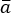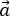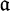## Equation in Word 2016 365 2013 2010 2007 2003

### How to create vectors in Word

A vector is a mathematical object characterized by magnitude and direction. To define a vector, use a bar above (), an arrow above (), bold or gothic font ().

### Insert a vertical bar with integration limits into the equation

For some standards, it is necessary to add to the equation the vertical bar with integration limits. Microsoft Word doesn't propose the simple way how to add it.

### How to create captions for equations

When you add formulas to your document, it may need to add captions - auto-numbered on the same line as equation right justified. It is easy to add captions for the equation:

### How to insert an equation with a system of linear equations or linear system

This tip shows how to add an equation with a system of linear equations (or linear system) – a collection of two or more linear equations involving the same set of variables.

### How to insert an equation with trigonometric functions

This tip shows how to add an equation with trigonometric functions, for example, the Euler's formula.

### How to insert an equation with a limit

This tip shows how to add an equation with a limit. For example, the formula of a limit.

### How to insert a Boolean algebra equation

This tip shows how to insert a Boolean algebra equation, for example, one of De Morgan's laws.

### How to quickly insert Script symbols in Word equations

In mathematics often is used a script Latin alphabet. This tip is about quick way how to paste letters in Script in your Microsoft Word document.

### How to quickly insert Fraktur symbols in Word equations

Individual Fraktur letters are sometimes used in mathematics. This tip is about quick way how to paste letters in Fraktur in your document Microsoft Word.

### How to quickly insert Double strike or Blackboard bold symbols in Word equations

Double strike or Blackboard bold is a typeface style that is often used for certain symbols in mathematical texts, in which certain lines of the symbol (usually vertical or near-vertical lines) are doubled. The symbols usually denote number sets (see some of usual symbols below).